# 神经网络学习

Posted by jjx on December 5, 2016

K 类分类:$S_L$=K, $y_i$ = 1 表示分到第 i 类;(K>2)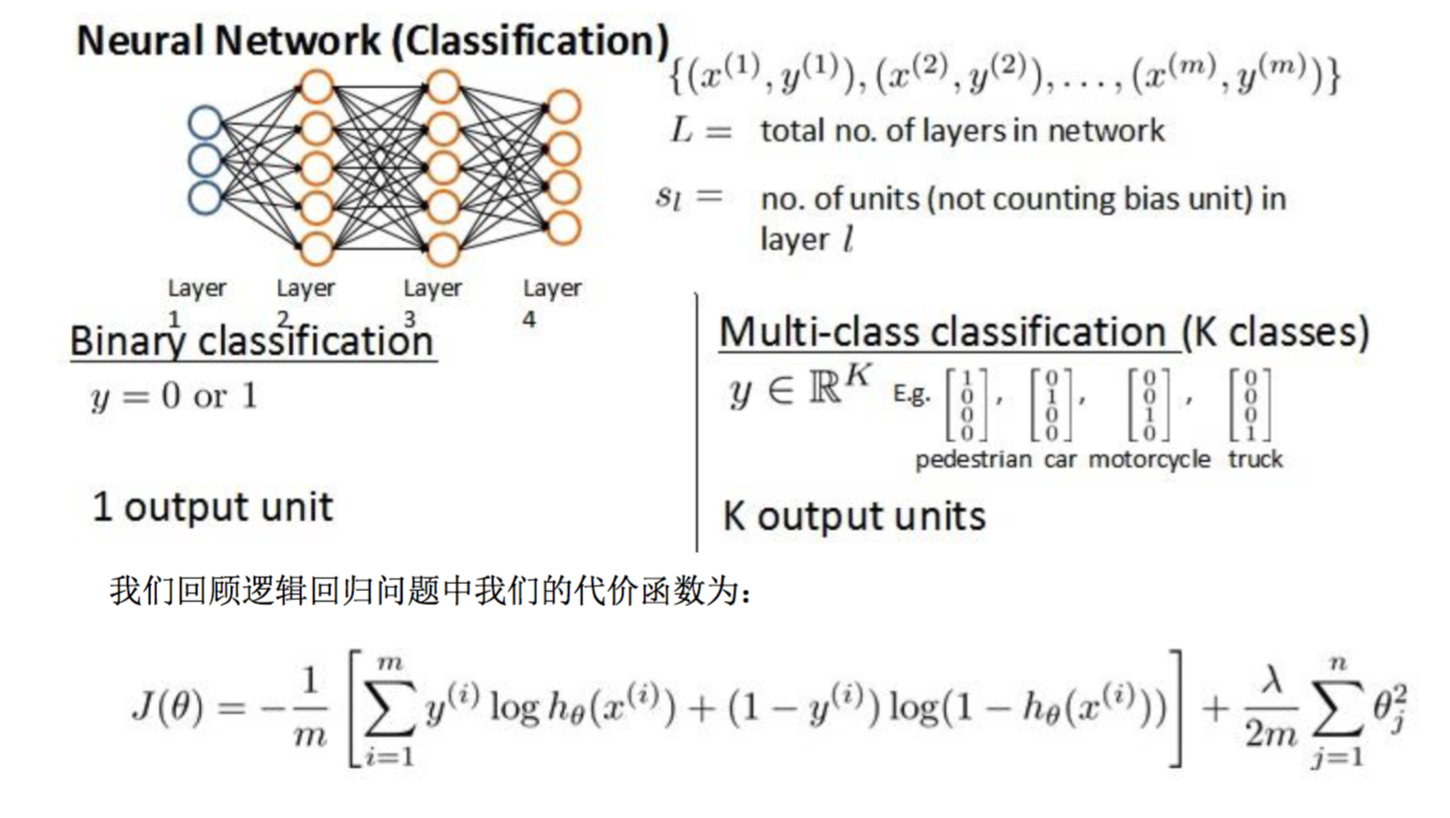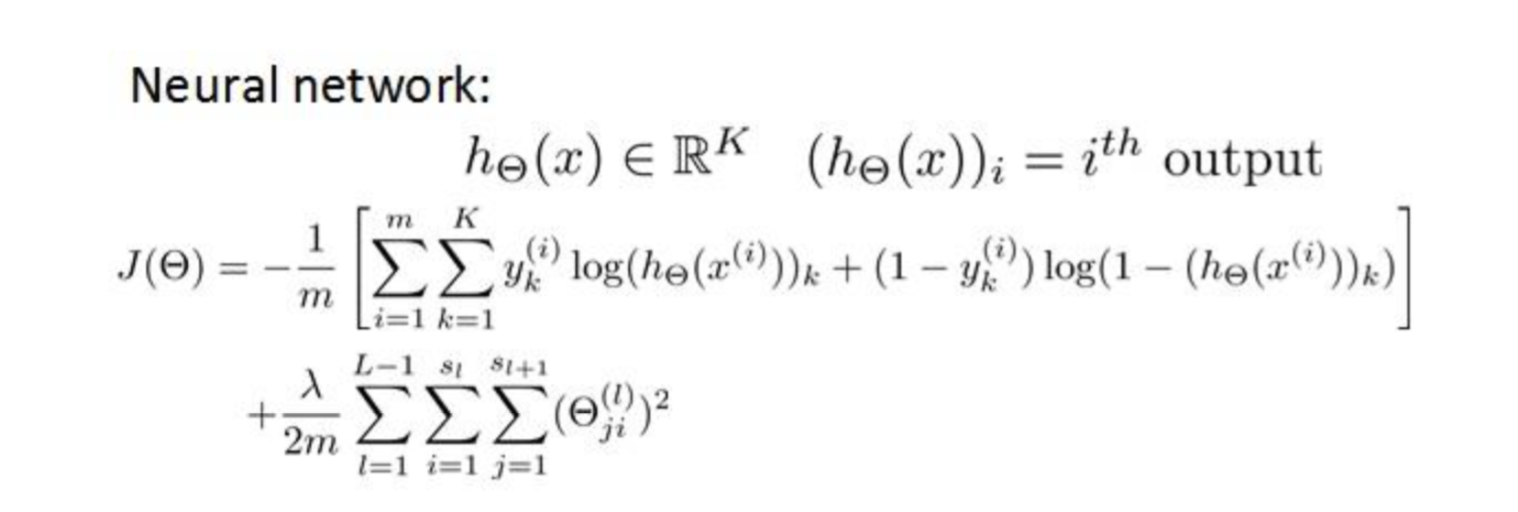#### 反向传播算法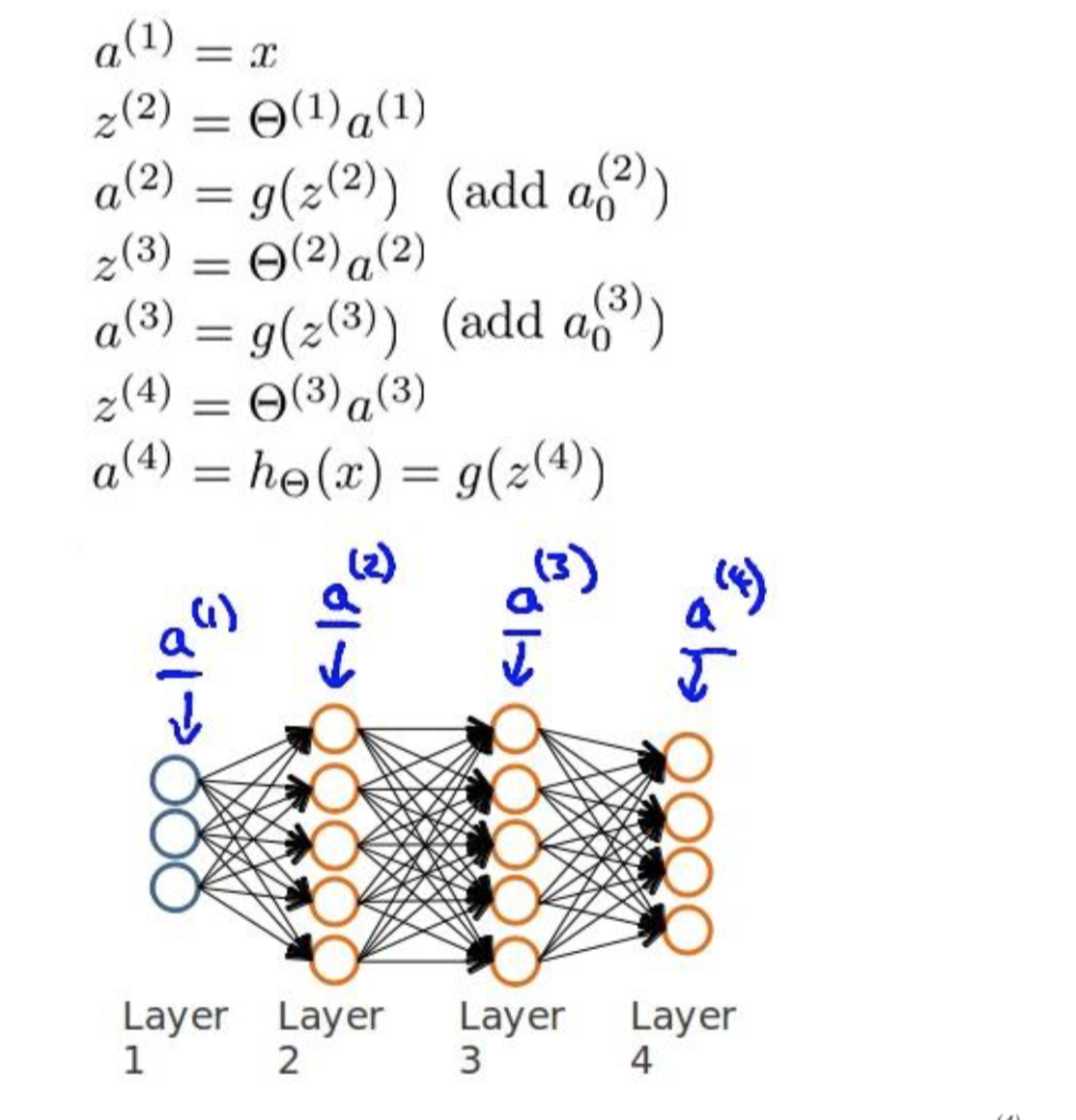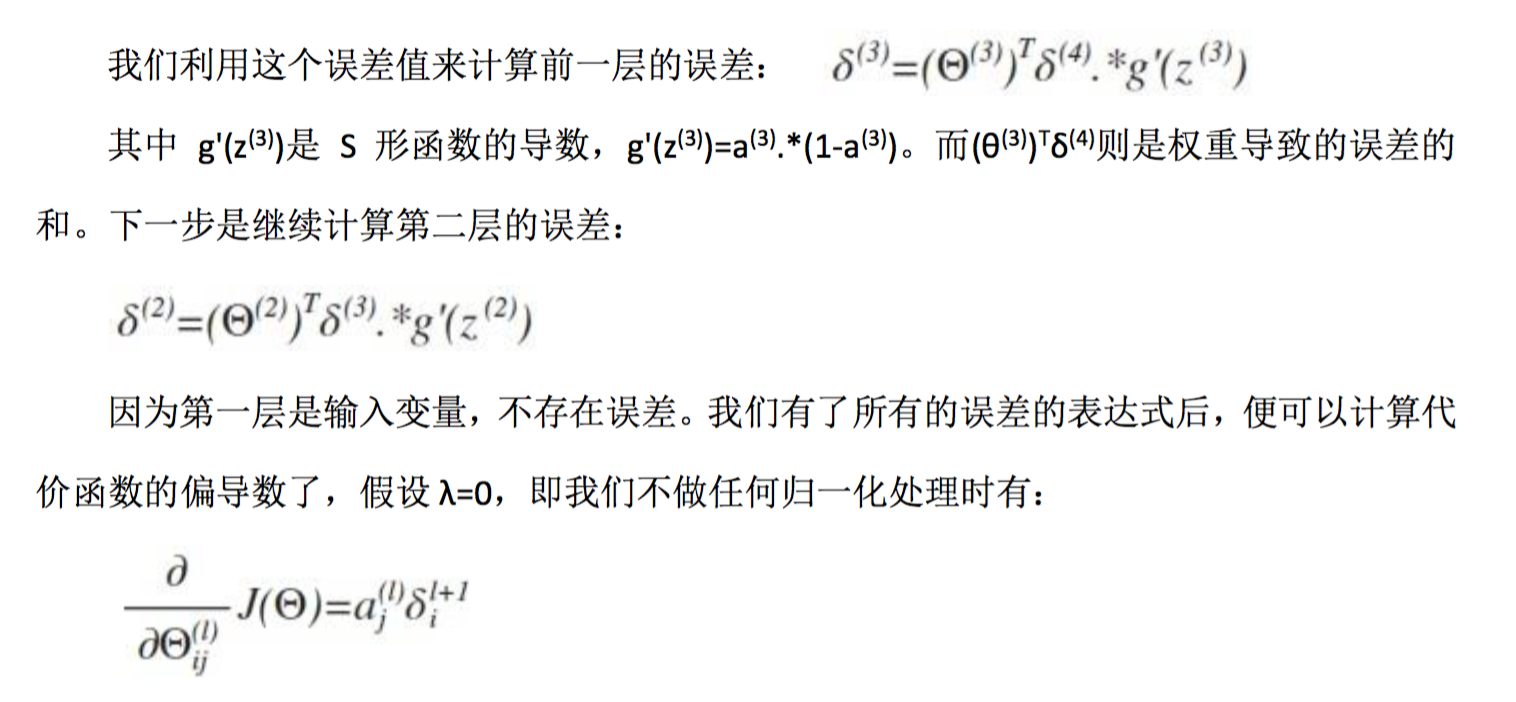$\delta^{(l)}_j=\frac{\sigma}{\sigma(z^{(l)}_j)}J(\theta)$

l 代表目前所计算的是第几层
j 代表目前计算层中的激活单元的下标,也将是下一层的第 j 个输入变量的下标。
i 代表下一层中误差单元的下标,是受到权重矩阵中第 i 行影响的下一层中的误差单元的下标。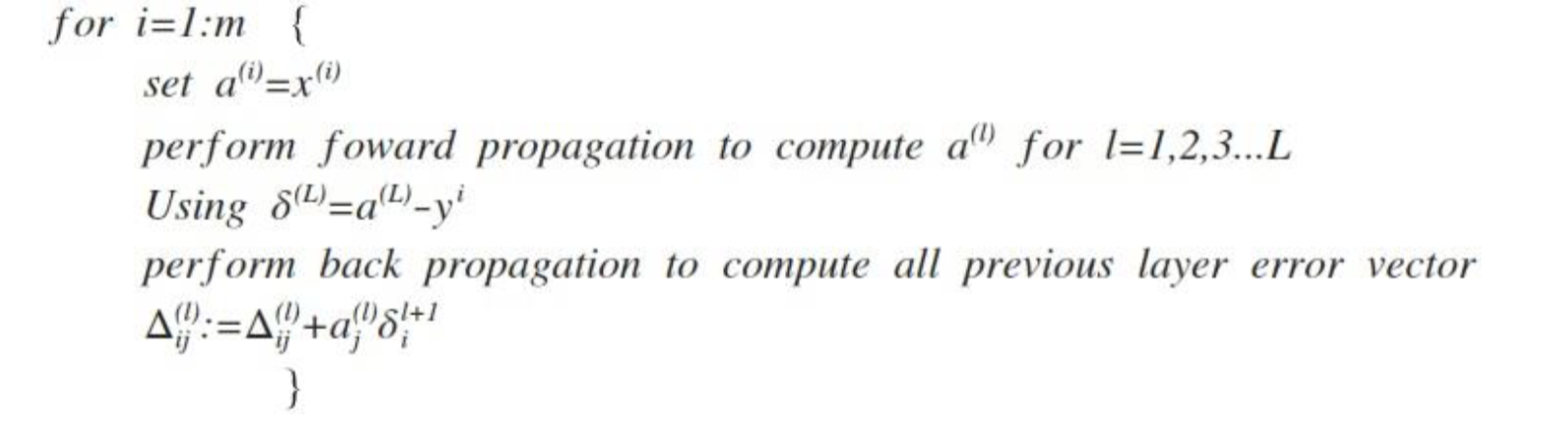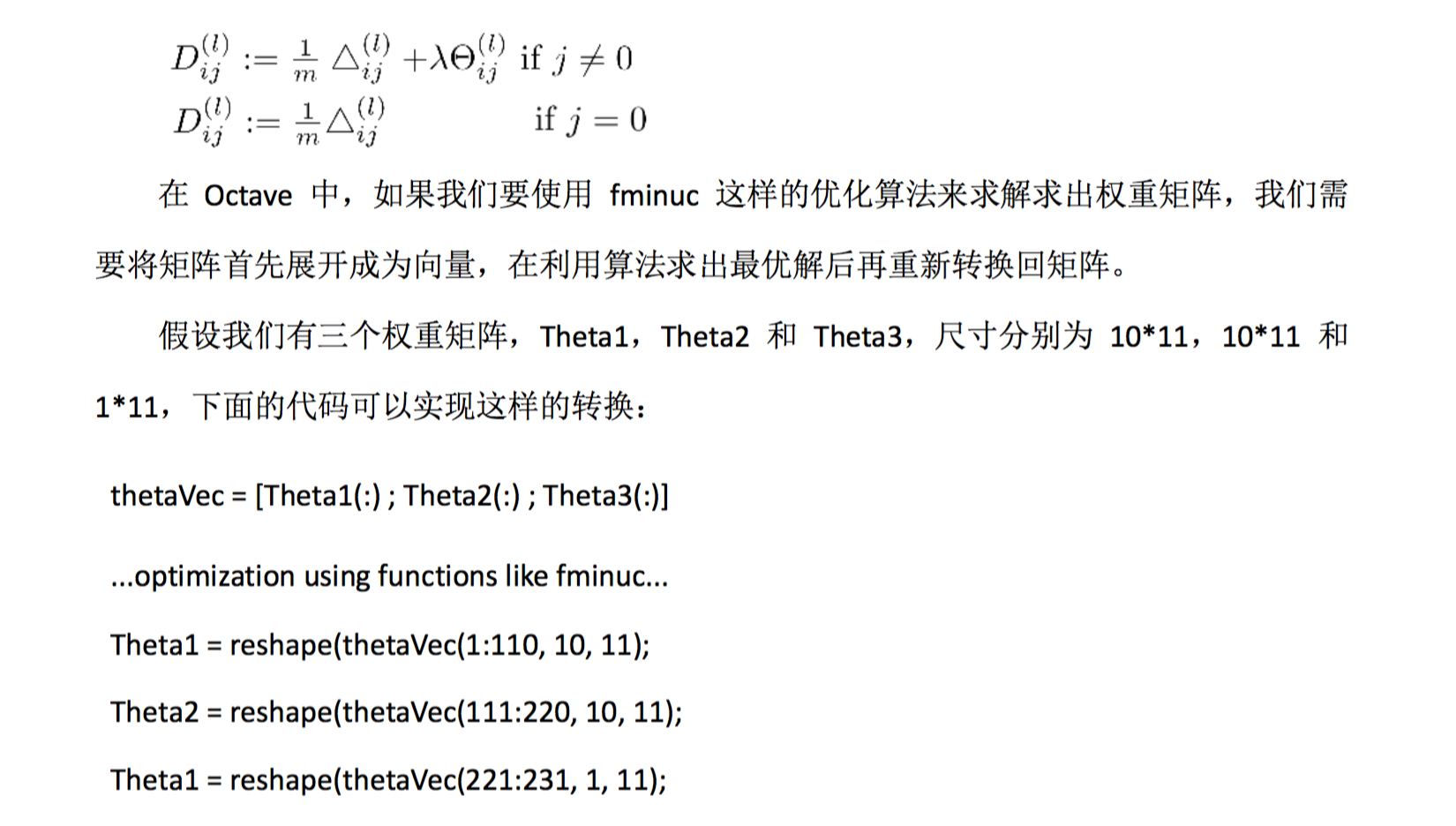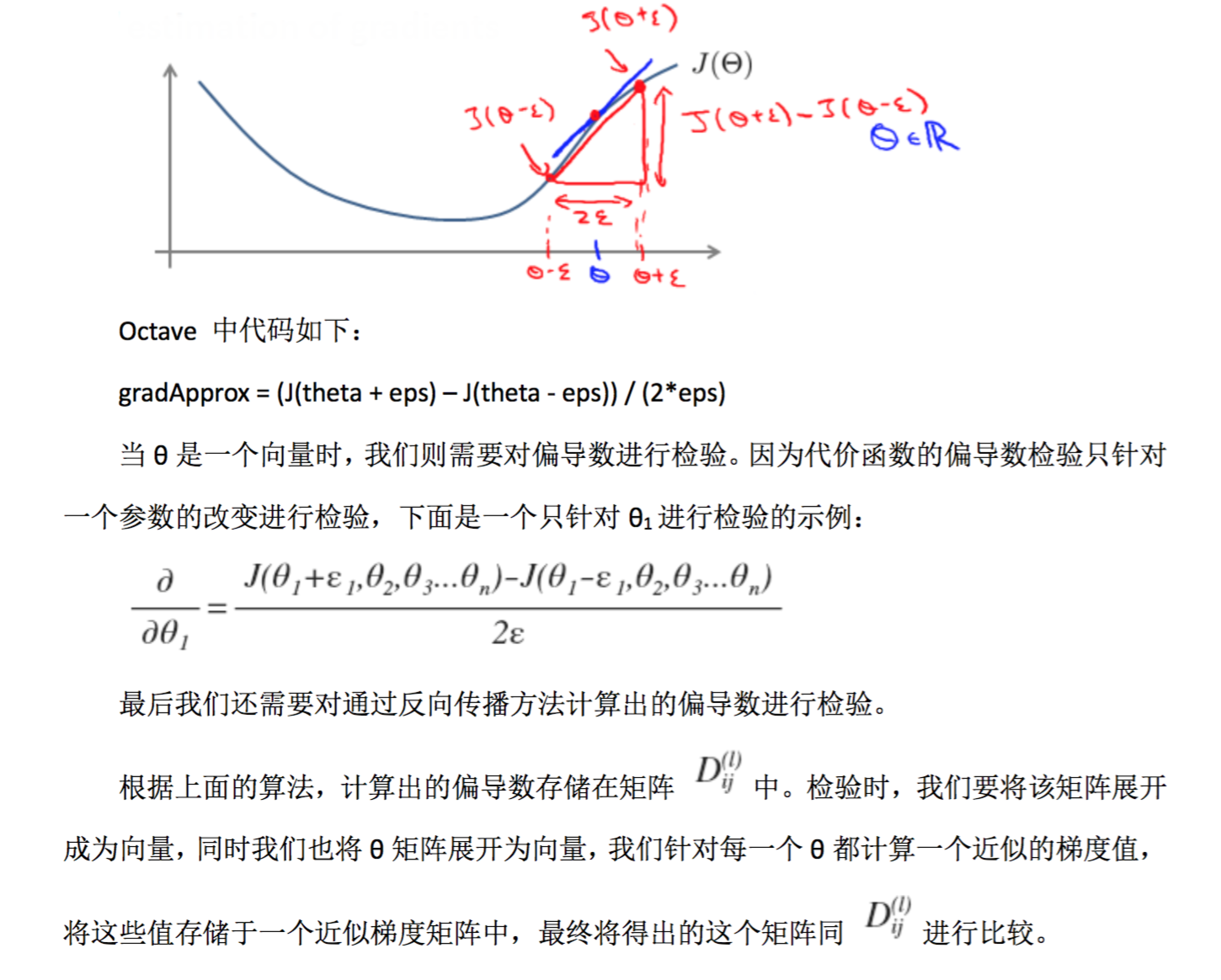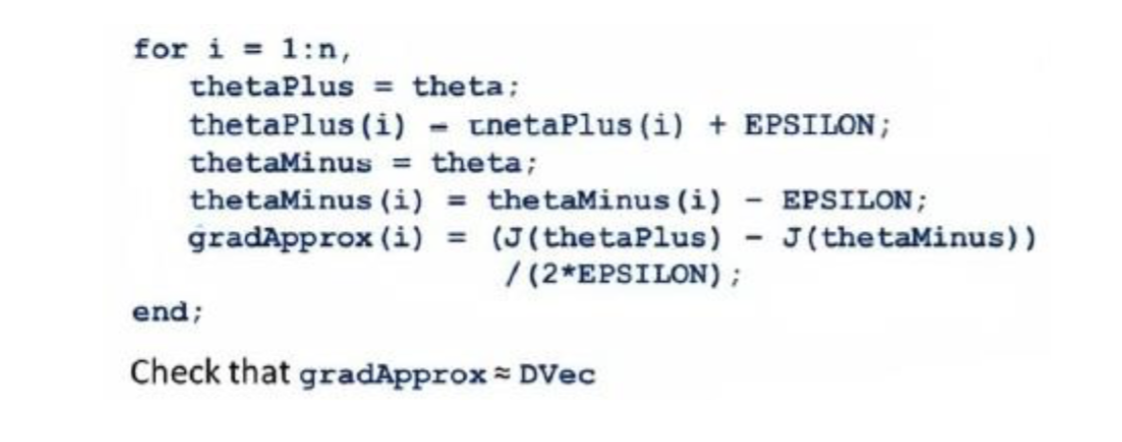Theta1 = rand(10, 11) * (2*eps) – eps

1. 参数的随机初始化
2. 利用正向传播方法计算所有的 hθ(x)
3. 编写计算代价函数 J 的代码
4. 利用反向传播方法计算所有偏导数
5. 利用数值检验方法检验这些偏导数
6. 使用优化算法来最小化代价函数